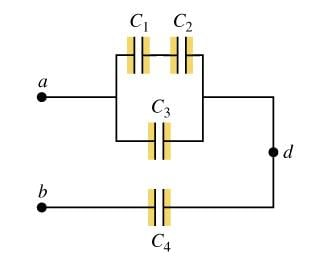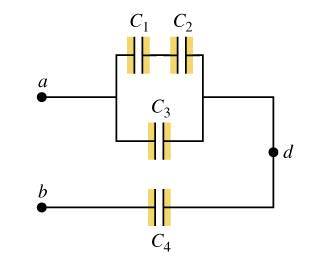# Capacitors in a circuit

## Homework StatementEach capacitor is $$5 \mu F$$ and $$V_{ab} = 30 V$$. I'm trying to find out the charge on each capacitor.

## Homework Equations

$$C = \frac QV$$
$$C_{eq} = C_1 + C_2 +...$$ for capacitors in parallel.
$$C_{eq} = \frac{1}{\frac{1}{C_1}+\frac{1}{C_2}+...}$$ for capacitors in series.

## The Attempt at a Solution

The first thing I did was calculate the equivalent capacitance for the entire circuit to be 3 microfarads. From here I calculate the total amount of charge to be 9*10^-5 C. Then I consider the circuit with just $$C_4$$ and the equivalent capacitance of capacitors 1-3. It's just two capacitors in series, and charge should be equally distributed between capacitors in series, right? So I get $$C_4 = 4.5 * 10^{-5} C$$, but this is incorrect. What am I doing wrong?

## Homework StatementEach capacitor is $$5 \mu F$$ and $$V_{ab} = 30 V$$. I'm trying to find out the charge on each capacitor.

## Homework Equations

$$C = \frac QV$$
$$C_{eq} = C_1 + C_2 +...$$ for capacitors in parallel.
$$C_{eq} = \frac{1}{\frac{1}{C_1}+\frac{1}{C_2}+...}$$ for capacitors in series.

## The Attempt at a Solution

The first thing I did was calculate the equivalent capacitance for the entire circuit to be 3 microfarads. From here I calculate the total amount of charge to be 9*10^-5 C. Then I consider the circuit with just $$C_4$$ and the equivalent capacitance of capacitors 1-3. It's just two capacitors in series, and charge should be equally distributed between capacitors in series, right? So I get $$C_4 = 4.5 * 10^{-5} C$$, but this is incorrect. What am I doing wrong?
Both capacitors in series would have the 9 and not the 4.5. To understand this realize that a capacitor is a break in the circuit. Current (and therefore charge) do not cross over that break. Instead, one plate has charge moving onto it while the other plate has charge moving off of it. The result is each plate gains a charge equal in magnitude and opposite in sign while current flows in the circuit. It is easy to forget after seeing current that no current actually flows through the capacitor.

In the series connection, the total charge drawn from the battery to the capacitor c(1234) must therefore rest on the first plate it comes in contact with. We have C(123) and C(4). Using this logic -- since again there is a break in the circuit -- all of the charge stores from the applied voltage must be on C(123). Where then, you may ask, does C(4) get its charge? I point finger to the C(123)'s bottom plate whose charge leaves it and therefore heads toward C(4)'s top plate. Then, C(4)'s top plate accepts that charge -- which is equal to the charge on C(123)'s top & bottom plate(read: that is, the total charge of 9). Then, C(4)'s bottom plate has charge leave it, creating current until eventually it too has 9.

Remember, for plates that are parallel, charge is shared by them.

Last edited:
Okay, that makes sense. Thanks for the explanation; I managed to figure out the rest with your help.